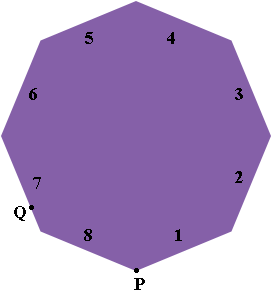SEARCH HOMEMath Central Quandaries & QueriesQuestion from Priscilla, a student: What side of an octagon is a snail on if it is 17/20 way around? I think it is 6.8? Does that mean it is on side 6 or 7?Hi Priscilla,

Assuming you mean a regular octagon I drew a diagram.If you start at P and go counterclockwise around the regular octagon until you are $\frac{17}{20}$ of the way around then, $\frac{17}{20} \times 8 = 6.8,$ so you are at $Q$ which is on the side numbered 7.

I hope this helps,
PennyMath Central is supported by the University of Regina and The Pacific Institute for the Mathematical Sciences.